Anzeige

# 07 - PPT - reversible reactions NJT modified.pptx

25. Mar 2023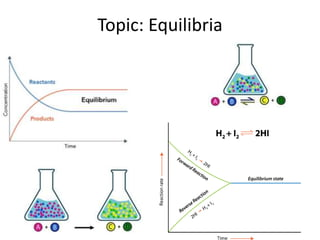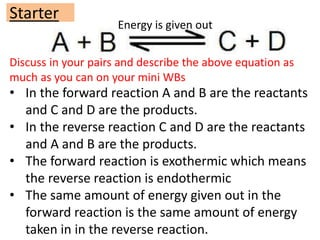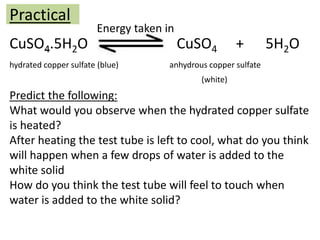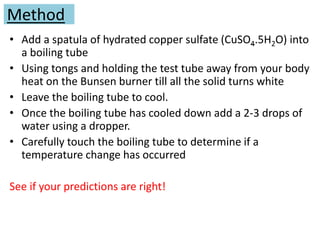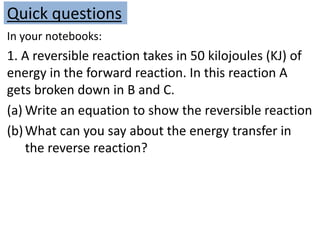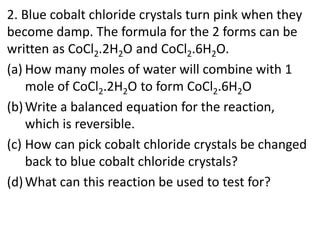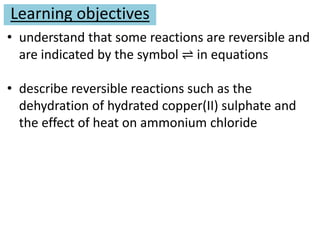1 von 7
Anzeige

### 07 - PPT - reversible reactions NJT modified.pptx

1. Topic: Equilibria
2. Discuss in your pairs and describe the above equation as much as you can on your mini WBs Starter • In the forward reaction A and B are the reactants and C and D are the products. • In the reverse reaction C and D are the reactants and A and B are the products. • The forward reaction is exothermic which means the reverse reaction is endothermic • The same amount of energy given out in the forward reaction is the same amount of energy taken in in the reverse reaction. Energy is given out
3. Practical CuSO4.5H2O CuSO4 + 5H2O hydrated copper sulfate (blue) anhydrous copper sulfate (white) Energy taken in Predict the following: What would you observe when the hydrated copper sulfate is heated? After heating the test tube is left to cool, what do you think will happen when a few drops of water is added to the white solid How do you think the test tube will feel to touch when water is added to the white solid?
4. Method • Add a spatula of hydrated copper sulfate (CuSO4.5H2O) into a boiling tube • Using tongs and holding the test tube away from your body heat on the Bunsen burner till all the solid turns white • Leave the boiling tube to cool. • Once the boiling tube has cooled down add a 2-3 drops of water using a dropper. • Carefully touch the boiling tube to determine if a temperature change has occurred See if your predictions are right!
5. Quick questions In your notebooks: 1. A reversible reaction takes in 50 kilojoules (KJ) of energy in the forward reaction. In this reaction A gets broken down in B and C. (a) Write an equation to show the reversible reaction (b)What can you say about the energy transfer in the reverse reaction?
6. 2. Blue cobalt chloride crystals turn pink when they become damp. The formula for the 2 forms can be written as CoCl2.2H2O and CoCl2.6H2O. (a) How many moles of water will combine with 1 mole of CoCl2.2H2O to form CoCl2.6H2O (b)Write a balanced equation for the reaction, which is reversible. (c) How can pick cobalt chloride crystals be changed back to blue cobalt chloride crystals? (d)What can this reaction be used to test for?
7. Learning objectives • understand that some reactions are reversible and are indicated by the symbol ⇌ in equations • describe reversible reactions such as the dehydration of hydrated copper(II) sulphate and the effect of heat on ammonium chloride
Anzeige